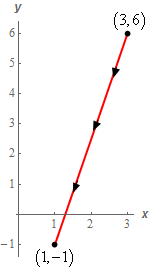Paul's Online Notes
Home / Calculus III / Line Integrals / Line Integrals - Part I
Show Mobile Notice Show All Notes Hide All Notes
Mobile Notice
You appear to be on a device with a "narrow" screen width (i.e. you are probably on a mobile phone). Due to the nature of the mathematics on this site it is best views in landscape mode. If your device is not in landscape mode many of the equations will run off the side of your device (should be able to scroll to see them) and some of the menu items will be cut off due to the narrow screen width.

### Section 16.2 : Line Integrals - Part I

1. Evaluate $$\displaystyle \int\limits_{C}{{3{x^2} - 2y\,ds}}$$ where $$C$$ is the line segment from $$\left( {3,6} \right)$$ to $$\left( {1, - 1} \right)$$.

Show All Steps Hide All Steps

Start Solution

Here is a quick sketch of $$C$$ with the direction specified in the problem statement shown.Show Step 2

Now, with the specified direction we can see that $$x$$ is decreasing as we move along the curve in the specified direction. This means that we can’t just determine the equation of the line and use that to work the problem. Using the equation of the line would require us to use increasing $$x$$ since the limits in the integral must go from smaller to larger value.

We could of course use the fact from the notes that relates the line integral with a specified direction and the line integral with the opposite direction to allow us to use the equation of the line. However, for this problem let’s just work with problem without the fact to make sure we can do that type of problem

So, we’ll need to parameterize this line and we know how to parameterize the equation of a line between two points. Here is the vector form of the parameterization of the line.

$\vec r\left( t \right) = \left( {1 - t} \right)\left\langle {3,6} \right\rangle + t\left\langle {1, - 1} \right\rangle = \left\langle {3 - 2t,6 - 7t} \right\rangle \,\,\,\,\,\,\,\,0 \le t \le 1$

We could also break this up into parameter form as follows.

$\begin{array}{*{20}{l}}{x = 3 - 2t}\\{y = 6 - 7t}\end{array}\hspace{0.25in}0 \le t \le 1$

Either form of the parameterization will work for the problem but we’ll use the vector form for the rest of this problem.

Show Step 3

We’ll need the magnitude of the derivative of the parameterization so let’s get that.

$\vec r'\left( t \right) = \left\langle { - 2, - 7} \right\rangle \hspace{0.25in}\hspace{0.25in}\left\| {\vec r'\left( t \right)} \right\| = \sqrt {{{\left( { - 2} \right)}^2} + {{\left( { - 7} \right)}^2}} = \sqrt {53}$

We’ll also need the integrand “evaluated” at the parameterization. Recall all this means is we replace the $$x$$/$$y$$ in the integrand with the $$x$$/$$y$$ from parameterization. Here is the integrand evaluated at the parameterization.

$3{x^2} - 2y = 3{\left( {3 - 2t} \right)^2} - 2\left( {6 - 7t} \right) = 3{\left( {3 - 2t} \right)^2} - 12 + 14t$ Show Step 4

The line integral is then,

\begin{align*}\int\limits_{C}{{3{x^2} - 2y\,ds}} & = \int_{0}^{1}{{\left( {3{{\left( {3 - 2t} \right)}^2} - 12 + 14t} \right)\sqrt {53} \,dt}}\\ & = \sqrt {53} \left. {\left[ { - \frac{1}{2}{{\left( {3 - 2t} \right)}^3} - 12t + 7{t^2}} \right]} \right|_0^1 = \require{bbox} \bbox[2pt,border:1px solid black]{{8\sqrt {53} }}\end{align*}

Note that we didn’t multiply out the first term in the integrand as we could do a quick substitution to do the integral.